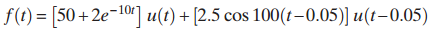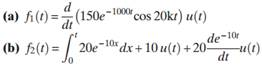### Create an Account

Already have account?

### Forgot Your Password ?

Home / Questions / Use MATLAB to find the Laplace transform of the following waveform Find the Laplace tra...

# Use MATLAB to find the Laplace transform of the following waveform Find the Laplace transforms of the following waveforms

Use MATLAB to find the Laplace transform of the following waveform2. Find the Laplace transforms of the following waveforms.Jun 15 2020 View more View Less

#### Answer (Solved)Subscribe To Get Solution